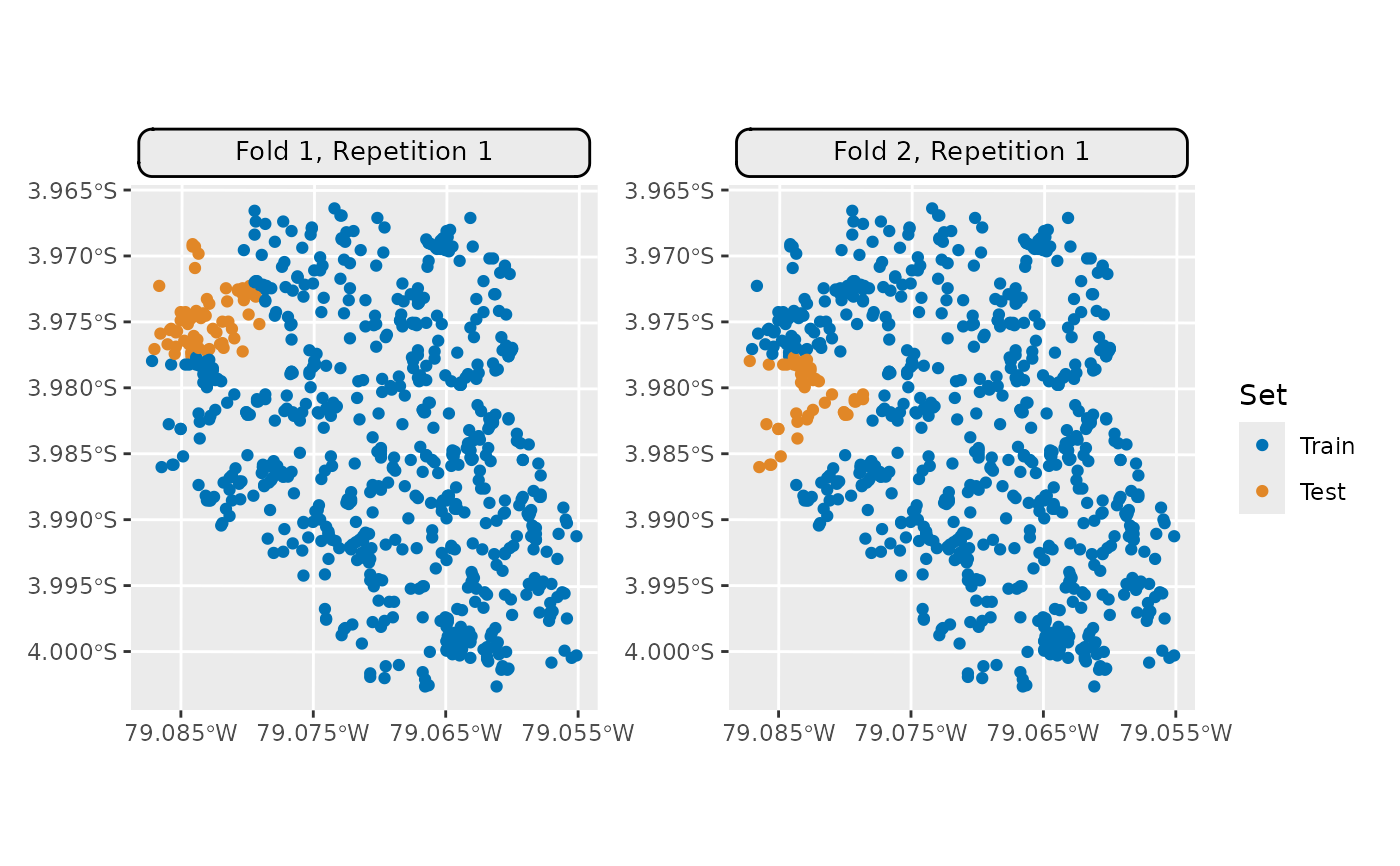Generic S3 plot() and autoplot() (ggplot2) methods.

## Usage

# S3 method for ResamplingSpCVCoords
autoplot(
object,
fold_id = NULL,
plot_as_grid = TRUE,
train_color = "#0072B5",
test_color = "#E18727",
sample_fold_n = NULL,
...
)

# S3 method for ResamplingRepeatedSpCVCoords
autoplot(
object,
fold_id = NULL,
repeats_id = 1,
plot_as_grid = TRUE,
train_color = "#0072B5",
test_color = "#E18727",
sample_fold_n = NULL,
...
)

# S3 method for ResamplingSpCVCoords
plot(x, ...)

# S3 method for ResamplingRepeatedSpCVCoords
plot(x, ...)

## Arguments

object

[Resampling]
mlr3 spatial resampling object of class ResamplingSpCVCoords or ResamplingRepeatedSpCVCoords.

[TaskClassifST]/[TaskRegrST]

fold_id

[numeric]
Fold IDs to plot.

plot_as_grid

[logical(1)]
Should a gridded plot using via patchwork be created? If FALSE a list with of ggplot2 objects is returned. Only applies if a numeric vector is passed to argument fold_id.

train_color

[character(1)]
The color to use for the training set observations.

test_color

[character(1)]
The color to use for the test set observations.

sample_fold_n

[integer]
Number of points in a random sample stratified over partitions. This argument aims to keep file sizes of resulting plots reasonable and reduce overplotting in dense datasets.

...

Passed to geom_sf(). Helpful for adjusting point sizes and shapes.

repeats_id

[numeric]
Repetition ID to plot.

x

[Resampling]
mlr3 spatial resampling object of class ResamplingSpCVCoords or ResamplingRepeatedSpCVCoords.

• mlr3book chapter on "Spatiotemporal Visualization"

• autoplot.ResamplingSpCVBlock()

• autoplot.ResamplingSpCVBuffer()

• autoplot.ResamplingSpCVEnv()

• autoplot.ResamplingSpCVDisc()

• autoplot.ResamplingSpCVTiles()

• autoplot.ResamplingCV()

• autoplot.ResamplingSptCVCstf()

## Examples

if (mlr3misc::require_namespaces(c("sf"), quietly = TRUE)) {
library(mlr3)
library(mlr3spatiotempcv)